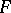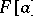# Algebraic algebra

(diff) ← Older revision | Latest revision (diff) | Newer revision → (diff)
An algebrawith associative powers (in particular, an associative algebra) over a fieldall elements of which are algebraic (an elementis called algebraic if the subalgebragenerated by it is finite-dimensional or, equivalently, if the elementhas an annihilating polynomial with coefficients from the ground field). An algebrais called an algebraic algebra of bounded degree if it is algebraic and if the set of degrees of the minimal annihilating polynomials of its elements is bounded. Subalgebras and homomorphic images of an algebraic algebra (of bounded degree) are algebraic algebras (of bounded degree).
The algebras considered below are associative. The Jacobson radical of an algebraic algebra is a nil-ideal. A primitive algebraic algebrais isomorphic to a dense algebra of linear transformations of a vector space over a skew-field; if, in addition,is of bounded degree, thenis isomorphic to a ring of matrices over a skew-field. An algebraic algebra without non-zero nilpotent elements (in particular, a skew-field) over a finite field is commutative. It follows that finite skew-fields are commutative. An algebraic algebra of bounded degree satisfies a polynomial identity (cf. PI-algebra). An algebraic PI-algebra is locally finite. If the ground field is uncountable, then the algebras obtained from an algebraic algebra by extension of the ground field, and the tensor product of algebraic algebras, are algebraic algebras.